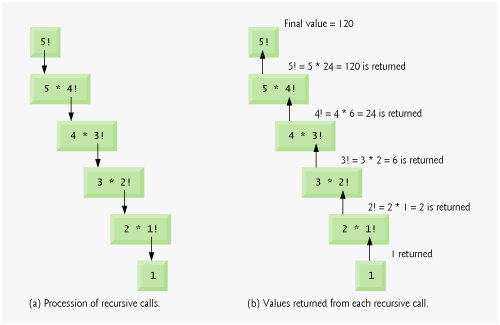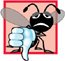# Recursion

The programs we have discussed are generally structured as functions that call one another in a disciplined, hierarchical manner. For some problems, it is useful to have functions call themselves. A recursive function is a function that calls itself, either directly, or indirectly (through another function). Recursion is an important topic discussed at length in upper-level computer science courses. This section and the next present simple examples of recursion. This book contains an extensive treatment of recursion. Figure 6.33 (at the end of Section 6.21) summarizes the recursion examples and exercises in the book.

 Although many compilers allow function main to call itself, Section 3.6.1, paragraph 3, of the C++ standard document indicates that main should not be called within a program. Its sole purpose is to be the starting point for program execution.

We first consider recursion conceptually, then examine two programs containing recursive functions. Recursive problem-solving approaches have a number of elements in common. A recursive function is called to solve a problem. The function actually knows how to solve only the simplest case(s), or so-called base case(s). If the function is called with a base case, the function simply returns a result. If the function is called with a more complex problem, it typically divides the problem into two conceptual piecesa piece that the function knows how to do and a piece that it does not know how to do. To make recursion feasible, the latter piece must resemble the original problem, but be a slightly simpler or slightly smaller version. This new problem looks like the original problem, so the function launches (calls) a fresh copy of itself to work on the smaller problemthis is referred to as a recursive call and is also called the recursion step. The recursion step often includes the keyword return, because its result will be combined with the portion of the problem the function knew how to solve to form a result that will be passed back to the original caller, possibly main.

The recursion step executes while the original call to the function is still open, i.e., it has not yet finished executing. The recursion step can result in many more such recursive calls, as the function keeps dividing each new subproblem with which the function is called into two conceptual pieces. In order for the recursion to eventually terminate, each time the function calls itself with a slightly simpler version of the original problem, this sequence of smaller and smaller problems must eventually converge on the base case. At that point, the function recognizes the base case and returns a result to the previous copy of the function, and a sequence of returns ensues all the way up the line until the original function call eventually returns the final result to main. All of this sounds quite exotic compared to the kind of "conventional" problem solving we have been using to this point. As an example of these concepts at work, let us write a recursive program to perform a popular mathematical calculation.

The factorial of a nonnegative integer n, written n! (and pronounced "n factorial"), is the product

n · ( n 1) · ( n 2) · ... · 1

with 1! equal to 1, and 0! defined to be 1. For example, 5! is the product 5 · 4 · 3 · 2 · 1, which is equal to 120.

The factorial of an integer, number, greater than or equal to 0, can be calculated iteratively (nonrecursively) by using a for statement as follows:

``` factorial = 1;

for ( int counter = number; counter >= 1; counter-- )
factorial *= counter;
```

A recursive definition of the factorial function is arrived at by observing the following relationship:

n! = n · ( n 1)!

For example, 5! is clearly equal to 5 * 4! as is shown by the following:

5! = 5 · 4 · 3 · 2 · 1

5! = 5 · (4 · 3 · 2 · 1)

5! = 5 · (4!)

The evaluation of 5! would proceed as shown in Fig. 6.28. Figure 6.28(a) shows how the succession of recursive calls proceeds until 1! is evaluated to be 1, which terminates the recursion. Figure 6.28(b) shows the values returned from each recursive call to its caller until the final value is calculated and returned.

Figure 6.28. Recursive evaluation of 5!.The program of Fig. 6.29 uses recursion to calculate and print the factorials of the integers 010. (The choice of the data type unsigned long is explained momentarily.) The recursive function factorial (lines 2329) first determines whether the terminating condition number <= 1 (line 25) is true. If number is indeed less than or equal to 1, function factorial returns 1 (line 26), no further recursion is necessary and the function terminates. If number is greater than 1, line 28 expresses the problem as the product of number and a recursive call to factorial evaluating the factorial of number - 1. Note that factorial ( number - 1 ) is a slightly simpler problem than the original calculation factorial ( number ).

Figure 6.29. Demonstrating function factorial.

(This item is displayed on page 291 in the print version)

``` 1 // Fig. 6.29: fig06_29.cpp
2 // Testing the recursive factorial function.
3 #include
4 using std::cout;
5 using std::endl;
6
7 #include
8 using std::setw;
9
10 unsigned long factorial( unsigned long ); // function prototype
11
12 int main()
13 {
14 // calculate the factorials of 0 through 10
15 for ( int counter = 0; counter <= 10; counter++ )
16 cout << setw( 2 ) << counter << "! = " << factorial( counter )
17 << endl;
18
19 return 0; // indicates successful termination
20 } // end main
21
22 // recursive definition of function factorial
23 unsigned long factorial( unsigned long number )
24 {
25  if ( number <= 1 ) // test for base case
26  return 1; // base cases: 0! = 1 and 1! = 1
27  else // recursion step
28  return number * factorial( number - 1 );
29 } // end function factorial
```

 ``` 0! = 1 1! = 1 2! = 2 3! = 6 4! = 24 5! = 120 6! = 720 7! = 5040 8! = 40320 9! = 362880 10! = 3628800 ```

Function factorial has been declared to receive a parameter of type unsigned long and return a result of type unsigned long. This is shorthand notation for unsigned long int. The C++ standard document requires that a variable of type unsigned long int be stored in at least four bytes (32 bits); thus, it can hold a value in the range 0 to at least 4294967295. (The data type long int is also stored in at least four bytes and can hold a value at least in the range 2147483648 to 2147483647.) As can be seen in Fig. 6.29, factorial values become large quickly. We chose the data type unsigned long so that the program can calculate factorials greater than 7! on computers with small (such as two-byte) integers. Unfortunately, function factorial produces large values so quickly that even unsigned long does not help us compute many factorial values before even the size of an unsigned long variable is exceeded.

The exercises explore using variables of data type double to calculate factorials of larger numbers. This points to a weakness in most programming languages, namely, that the languages are not easily extended to handle the unique requirements of various applications. As we will see when we discuss object-oriented programming in more depth, C++ is an extensible language that allows us to create classes that can represent arbitrarily large integers if we wish. Such classes already are available in popular class libraries, and we work on similar classes of our own in Exercise 9.14 and Exercise 11.5.

 Such classes can be found at shoup.net/ntl, cliodhna.cop.uop.edu/~hetrick/c-sources.html and www.trumphurst.com/cpplibs/datapage.phtml?category='intro'.

Common Programming Error 6.24Either omitting the base case, or writing the recursion step incorrectly so that it does not converge on the base case, causes "infinite" recursion, eventually exhausting memory. This is analogous to the problem of an infinite loop in an iterative (nonrecursive) solution.C++ How to Program (5th Edition)
ISBN: 0131857576
EAN: 2147483647
Year: 2004
Pages: 627